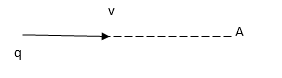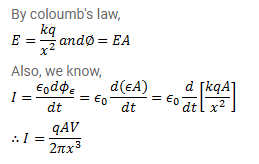# A point charge is moving along a straight line with a constant velocity v.

Question:

A point charge is moving along a straight line with a constant velocity v. Consider a small area A perpendicular to the direction of motion of the charge. Calculate the displacement current through the area when its distance from the charge is $x$. The value of $x$ is not large so that the electric field at any instant is essentially given by Coulomb's law.Solution: